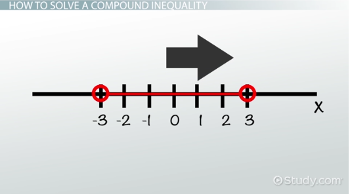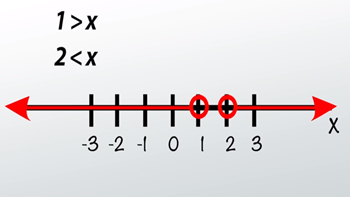# How to Solve 'And' & 'Or' Compound Inequalities

An error occurred trying to load this video.

Try refreshing the page, or contact customer support.

Coming up next: How to Solve and Graph One-Variable Inequalities

### You're on a roll. Keep up the good work!

Replay
Your next lesson will play in 10 seconds
• 0:05 What Are Inequalities?
• 2:21 How to Solve a…
• 4:44 Important Notes
• 6:35 Lesson Summary

Want to watch this again later?

Timeline
Autoplay
Autoplay
Speed

#### Recommended Lessons and Courses for You

Lesson Transcript
Instructor: Sarah Spitzig

Sarah has taught secondary math and English in three states, and is currently living and working in Ontario, Canada. She has recently earned a Master's degree.

In this lesson, we will learn the difference between a conjunction and a disjunction and how to solve and graph 'and' and 'or' inequalities on the number line.

## What Are Inequalities?

Sydney has an important math test coming up on Friday. She wants to study for her test for at least 30 minutes. Sydney's parents told her she needs to leave for swimming practice in 60 minutes. Therefore, she can study for no less than 30 minutes and no more than 60 minutes. This scenario can be written as a compound inequality. But what exactly does this mean?

You can think of an inequality as an equation, except that the equals sign is replaced with a less than or greater than sign. We still need to solve the inequality just like you would an equation. The only difference is that instead of one answer that makes the equation true, like x = 3, there are many answers that make an inequality true, like x < 5. In this case, all numbers less than five would make the inequality true.

• 2x + 5 = 7 is an equation because it has an equals sign.
• 2x + 5 < 7 is an inequality because it has an inequality sign.

A compound inequality is just more than one inequality that we want to solve at the same time. We can either use the word 'and' or 'or' to indicate if we are looking at the solution to both inequalities (and), or if we are looking at the solution to either one of the inequalities (or).

x < 7 and x > -3, which can also be written as -3 < x < 7, is a compound inequality because it is two inequalities connected by the word 'and'. This is also known as a conjunction. In this case, we are looking for the solution to both inequalities. In other words, this solution satisfies both inequalities.

x > 7 or x < -3 is a compound inequality, also known as a disjunction, because it is two inequalities connected by the word 'or'. In this case, we are looking for a solution to either one of the equations.

Let's check back in with Sydney. We know she needs to study for at least 30 minutes, but less than 60. If we set this up as a compound inequality, it looks like this: x > 30 and x < 60, also written as 30 < x < 60.

## How to Solve a Compound Inequality

#### Example 1

Let's take a look at the inequality 2 + x < 5 and -1 < 2 + x, which can also be written as -1 < 2 + x < 5. This is a compound inequality because it uses the word 'and.' Now let's go ahead and solve it.

1) Solve each part of the inequality separately.

2 + x < 5 and -1 < 2 + x

In the first equation, 2 + x < 5, we need to subtract 2 from each side to get the variable by itself. We then get x < 3.

In the second equation, -1 < 2 + x, we again subtract 2 from both sides. This gives us -3 < x.

Our solution, then, is x < 3 and -3 < x, or -3 < x < 3.

2) Graph on the number line.Since this is a conjunction, the space between -3 and 3 is where the answer lies. In other words, any value between -3 and 3 satisfies this compound inequality.

Remember Sydney? If we were to display her inequality on a number line, it would show that all numbers between 30 and 60 would be possible solutions. Meaning, she could study for 35, minutes, 42 minutes and so on.

#### Example 2

But what if we are solving a disjunction? Let's take a look at the following inequality: 7 > 2x + 5 or 7 < 5x - 3. This time the word 'or' is used instead of the word 'and'. How do we solve this?

1) Solve each inequality:

For 7 > 2x + 5, we subtract 5 from each side to get 2 > 2x. Divide each side by 2 and we get 1 > x.

For 7 < 5x - 3, we add 3 to each side and get 10 < 5x. Divide each side by 5 and we have 2 < x.

2) Graph on the number line.

Since this is a disjunction, any value greater than 2 and less than 1 is where the answer lies. All real numbers satisfy this compound inequality.## Important Notes

There are a few important points to keep in mind as you solve compound inequalities.

To unlock this lesson you must be a Study.com Member.

### Register to view this lesson

Are you a student or a teacher?

### Unlock Your Education

#### See for yourself why 30 million people use Study.com

##### Become a Study.com member and start learning now.
Back
What teachers are saying about Study.com

### Earning College Credit

Did you know… We have over 160 college courses that prepare you to earn credit by exam that is accepted by over 1,500 colleges and universities. You can test out of the first two years of college and save thousands off your degree. Anyone can earn credit-by-exam regardless of age or education level.